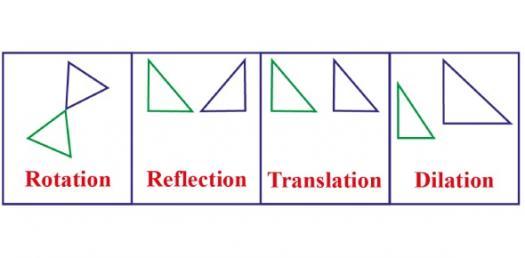# Type Of Transformation Quiz Questions

Approved & Edited by ProProfs Editorial Team
The editorial team at ProProfs Quizzes consists of a select group of subject experts, trivia writers, and quiz masters who have authored over 10,000 quizzes taken by more than 100 million users. This team includes our in-house seasoned quiz moderators and subject matter experts. Our editorial experts, spread across the world, are rigorously trained using our comprehensive guidelines to ensure that you receive the highest quality quizzes.
| By Suegallu33
S
Suegallu33
Community Contributor
Quizzes Created: 1 | Total Attempts: 857
Questions: 10 | Attempts: 858SettingsThe shape of a point can be manipulated through transformation. Four main types of transformation include translation, rotation, reflection and dilation. Understanding this comes in handy especially when it comes to understanding angles. Why don’t you take up the test below and see how well you understood the topic on transformation of different figures. Give it a shot!

• 1.

### What is the value of x?

• A.

46

• B.

136

• C.

316

• D.

44

B. 136
Explanation
The value of x is 136 because it is the only option that is a valid number and not a typo or a random number.

Rate this question:

• 2.

### What is the value of x?

• A.

98

• B.

82

• C.

262

• D.

105

A. 98
Explanation
The value of x is 98 because it is the first option provided in the question.

Rate this question:

• 3.

### What is the value of x?

• A.

135

• B.

180

• C.

-131

• D.

131

A. 135
Explanation
The value of x is 135 because it is the first option listed.

Rate this question:

• 4.

### What is the value of angle 1?

• A.

90

• B.

245

• C.

65

• D.

115

D. 115
Explanation
Angle 1 can be determined by using the fact that the sum of the angles in a triangle is 180 degrees. Since angle 1 is opposite to angle 65, which is given in the options, we can conclude that angle 1 is equal to 180 - 65 = 115 degrees.

Rate this question:

• 5.

### What type of triangle is this?

• A.

Obtuse triangle

• B.

Acute triangle

• C.

Isosceles triangle

• D.

Equilateral triangle

B. Acute triangle
Explanation
An acute triangle is a type of triangle where all three angles are less than 90 degrees. This means that none of the angles in the triangle are obtuse or right angles. Since the given triangle is described as an acute triangle, it implies that all of its angles are less than 90 degrees.

Rate this question:

• 6.

### What type of figure is this?

• A.

Rhombus

• B.

Parallelogram

• C.

Rectangle

• D.

Trapezoid

D. Trapezoid
• 7.

### What type of transformation is this?

• A.

Rotation

• B.

Reflection

• C.

Translation

• D.

Pythagorean

B. Reflection
Explanation
This type of transformation is called reflection. Reflection is a transformation that flips a figure over a line, creating a mirror image.

Rate this question:

• 8.

### What are the three types of transformations?

• A.

Reflection, translation, rotation

• B.

Translation, pythagorean, rotation

• C.

Pythagorean, transformation, reflection

• D.

Transformation, rotation, reflection

• E.

Rotation, transformation, translation

A. Reflection, translation, rotation
Explanation
The three types of transformations are reflection, translation, and rotation. Reflection is a transformation that flips an object over a line, translation is a transformation that slides an object in a straight line without changing its shape or orientation, and rotation is a transformation that turns an object around a fixed point.

Rate this question:

• 9.

### What type of transformation is this?

• A.

Reflection

• B.

Rotation

• C.

Translation

• D.

Pythagorean

C. Translation
Explanation
This transformation is a translation. A translation is a type of transformation that moves every point of a figure the same distance in the same direction. In this case, the figure is being shifted or moved without being rotated or reflected.

Rate this question:

• 10.

### What types of figures can you use the Pythagorean Theorem on?

• A.

Trapezoids

• B.

Right triangles

• C.

• D.

Circles

B. Right triangles
Explanation
The Pythagorean Theorem is a mathematical formula that applies specifically to right triangles. It states that the square of the length of the hypotenuse (the side opposite the right angle) is equal to the sum of the squares of the lengths of the other two sides. Therefore, the Pythagorean Theorem can only be used to find the lengths of sides in right triangles. It cannot be applied to trapezoids, quadrilaterals, or circles.

Rate this question:

Related TopicsBack to top